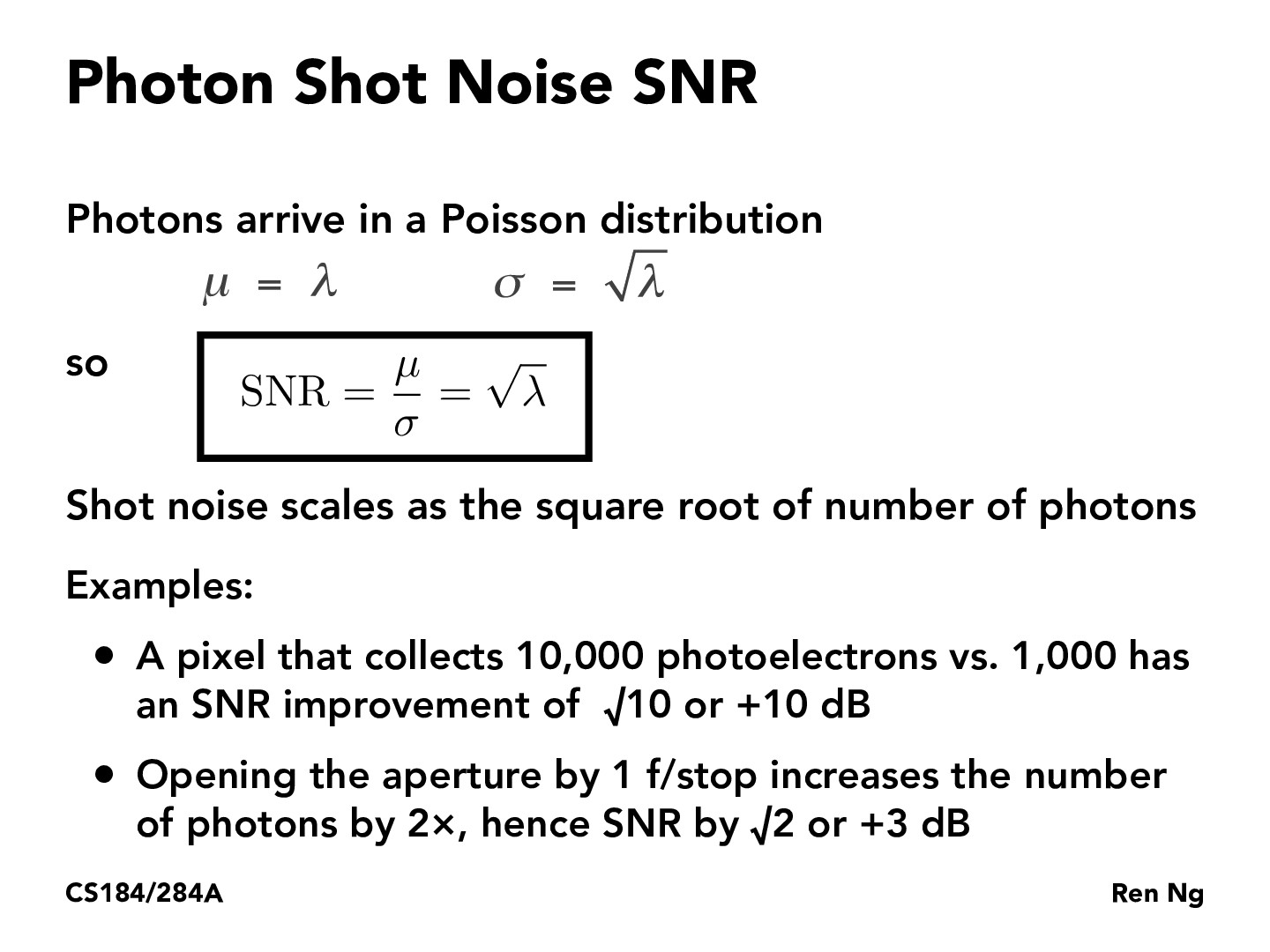You are viewing the course site for a past offering of this course. The current offering may be found here.Lecture 21: Image Sensors (79)tanmayghai18

Could someone explain the derivation of the Photon Shot Noise SNR in this slide please?ellenluo

We're using the equation described earlier with the specific values for a Poisson distribution. A Poisson distribution has a known mean rate lambda and it can be shown that its mean and variance are both equal to lambda. Since a standard deviation is the square root of the variance, we can substitute lambda and sqrt(lambda) into the SNR equation to get the shot noise SNR.kavimehta

Why do we care about the SNR? How does an increase or decrease effect an imagewjgan7

A higher SNR means less noise which means a less grainy image. I think the slide means that shot noise is inversely proportional to the square root of the number of photons?

You must be enrolled in the course to comment